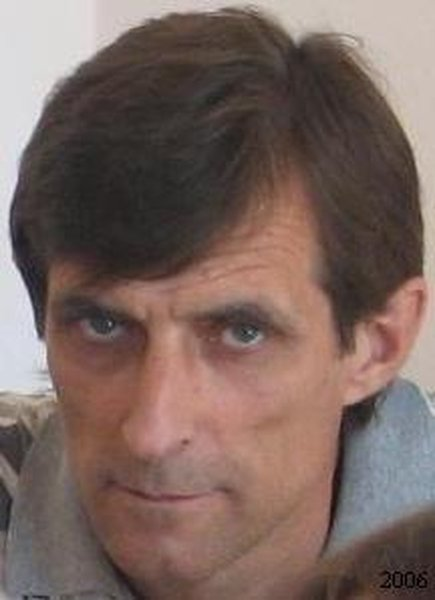Associate Professor, Leading Researcher

### Information

 Position: Associate Professor, Leading Researcher Doctor of Physical and Mathematical Science Senior Researcher 212 259-05-32 asymptotic methods, theory of averaging, equations of mathematical physics, mathematical hydrodynamics, variational problems, numerical mathematics, simulation, functional analysis

### Courses:

Modern problems of applied mathematics
Problems of non-classical optimization
Modern problems of mathematical physics

### Publications:

1. H.V. Sandrakov On some properties of solutions of Navier-Stokes equations with oscillating data. // J. Mathematical Sciences. – 2007. – V. 143, № 4. – p. 3377–338.